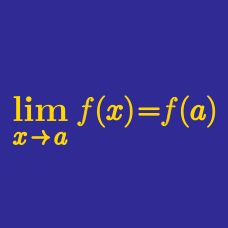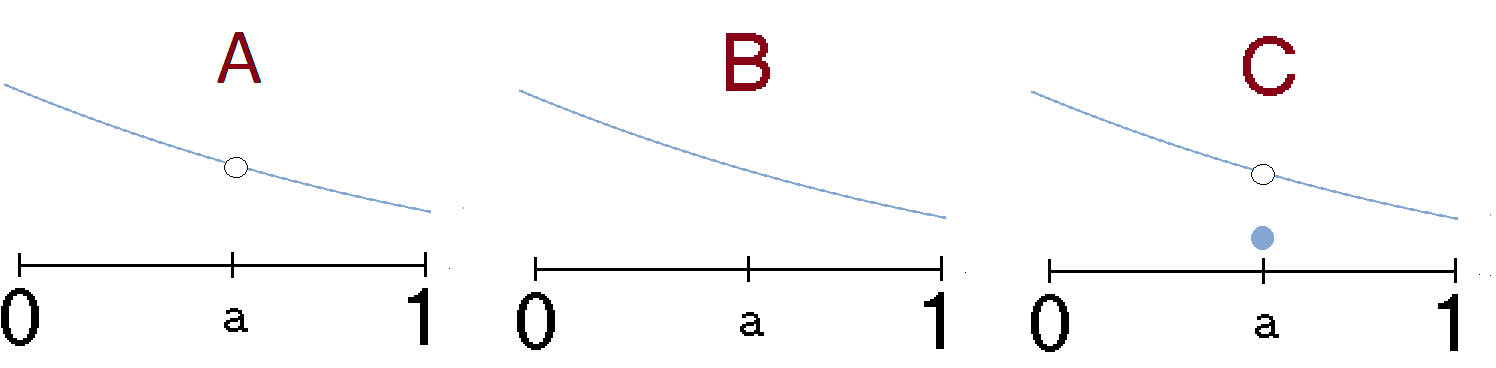Calculus

# Continuity WarmupWhich of the graphs show a function that is continous at $x=a?$

$g(x) = \begin{cases} \frac{1}{x} & \textrm{if } x \neq 0 \\ a & \textrm{if } x = 0 \\ \end{cases}$

Is there a real number $a$ for which the function $g(x)$ is continuous at $x = 0?$

$f(x)=\frac { x }{ x } ,g(x)=\frac { x^{ 2 } }{ x } ,h(x)=\frac { x }{ x^{ 2 } }$

Each of these functions is undefined at $x = 0.$ Which functions is it possible to extend by defining a functional value at $x = 0$ in such a way that the resulting extended function is continuous at $x = 0?$

True or False?

The function $f(x) = x^3 + x - 1$ has a root between 0 and 1.

  

Hint: $f(0) = -1$ and $f(1) = 1.$ Apply the Intermediate Value Theorem.Which of the graphs shows a function $f(x)$ for which $\lim_{x\to a} f(x)$ exists?

×